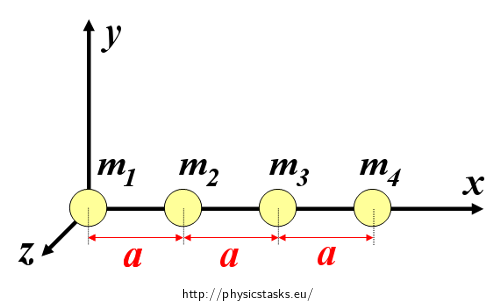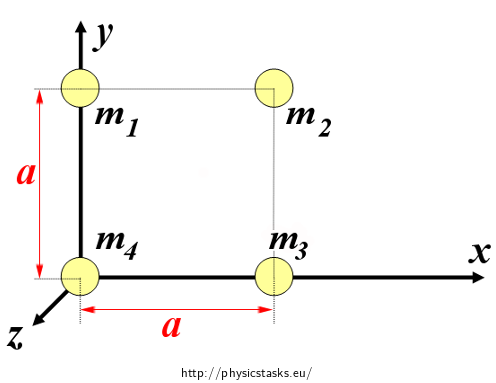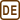## Centre of Mass of Variously Arranged Spheres

### Task number: 2232

Determine the centre of mass of a system which consists of four homogeneous spheres, whose masses are 1 kg, 2 kg, 3 kg a 4 kg respectively, if they are arranged as follows:

A) the spheres are located at equal distances on a straight line,
B) the spheres lie in the vertices of a square,
C) the spheres are in four adjacent vertices of a cube.

In all cases, distance between the centres of adjacent spheres is equal to a. In parts B) and C), the heaviest sphere lies in the origin of a coordinate system.

• #### Notation

 m1 = 1 kg mass of the 1st ball m2 = 2 kg mass of the 2nd ball m3 = 3 kg mass of the 3rd ball m4 = 4 kg mass of the 4th ball a distance between the centres of any two adjacent spheres rm = ? position of the centre of mass
• #### Hint 1 (to Part A)

Draw a picture capturing the situation and introduce a suitable coordinate system into it. Assign the corresponding coordinates in your system to the spheres' centres C1, C2, C3, C4.

• #### Hint 2 (to Part A)

Are you able to determine the y- and z-coordinate of the centre of mass right away?

• #### Hint 3 (to Part A)

Since the spheres' dimensions are not given, we can consider them to be point masses whose mass is concentrated in the centres of the spheres. This means that we can determine the position vector of the centre of mass using the centre of mass equation for a system of point masses. Do you know the equation? How will it change if we are only interested in the x-coordinate of the centre of mass?

• #### Hint 4 (to part A)

Itemize the sum in equation (1) and use the given values to calculate the coordinate xm.

• #### Hint 5 (to Part B)

Proceed the same way as in Part A - draw the described arrangement and place the spheres in a coordinate system (don't forget that the heaviest one is located in the origin of the coordinate system). Write the coordinates of the spheres' centres and think, whether one of the coordinates of the centre of mass can be determined right away.

• #### Hint 6 (to Part B)

To determine the x- and y-coordinate of the centre of mass use equation (1) again, and its variation for y-component. Use the given values and calculate both coordinates.

• #### Hint 7 (to part C)

In Part C, follow the same steps as before for the third time. Draw a picture, place the spheres into a coordinate system, determine the coordinates of their centres and use equation (1) and its variations (for other components) to determine xm, ym, zm.

• #### Comment

All the obtained results apply to the coordinate systems and spheres' location shown in the figures. If you considered different location of the spheres in the coordinate systems, your results would differ from the answer!

• #### Overall Solution

Since the spheres' dimensions are not given, we can consider them to be point masses whose mass is concentrated in the centres of the spheres. At the beginning of each part of the problem, we have to place the system of spheres into a suitable coordinate system.

Part A:

One possible implementation of a coordinate system and location of the spheres is shown in the figure below.In this coordinate system, centres of the spheres have the following coordinates:

$C_1\,=\,[0{,}0,0],$ $C_2\,=\,[a,0{,}0],$ $C_3\,=\,[2a,0{,}0],$ $C_4\,=\,[3a,0{,}0].$

Since the centres of all the spheres (and thus their centres of mass as well) lie on the x-axis, the centre of mass of the entire system will also lie on the x-axis. Each point of the x-axis has zero y- and z-coordinate:

$y_m\,=\,z_m\,=\,0.$

So, we just need to determine the x-coordinate of the centre of mass.

Formula for the position vector of the centre of mass of a system of n point masses is as follows:

$\vec{r}_m\,=\,\frac{\sum_{i=1}^n{m_i\vec{r}_i}}{\sum_{i=1}^n{m_i}},$

where mi are the masses of the individual point masses and ri the corresponding position vectors. Since the coordinates of the centre of mass can be determined separately, for the x-coordinate of the centre of mass of our system we have:

$x_m\,=\,\frac{\sum_{i=1}^4{m_ix_i}}{\sum_{i=1}^4{m_i}}.\tag{1}$

By itemizing the sum in equation (1), we get:

$x_m\,=\,\frac{m_1{\cdot}0\,+\,m_2{\cdot}a\,+\,m_3{\cdot}2a\,+\,m_4{\cdot}3a}{m_1\,+\,m_2\,+\,m_3\,+\,m_4}.\tag{2}$

Finally, we substitute the masses in relation (2):

$x_m\,=\,\frac{1{\cdot}0\,+\,2{\cdot}a\,+\,3{\cdot}2a\,+\,4{\cdot}3a}{1\,+\,2\,+\,3\,+\,4}\,=\,\frac{20a}{10}\,=\,2a.$

The centre of mass M of the system described in part A has the following coordinates:

$M\,=\,[2a,0{,}0].$

Part B:

One possible implementation of a coordinate system and location of the spheres is shown in the figure below.In this coordinate system, centres of the spheres have the following coordinates:

$C_1\,=\,[0,a,0],$ $C_2\,=\,[a,a,0],$ $C_3\,=\,[a,0{,}0],$ $C_4\,=\,[0{,}0,0].$

Since the centres of all spheres (and thus their centres of mass as well) lie in the xy-plane, the centre of mass of the entire system will also lie in the xy-plane. Each point of this plane has zero z-coordinate, thus for the centre of mass it it holds that:

$z_m\,=\,0.$

So, we just need to determine the x- and y-coordinate of the centre of mass.

According to equation (1), for the x-coordinate of the centre of mass xm we have:

$x_m\,=\,\frac{\sum_{i=1}^4{m_ix_i}}{\sum_{i=1}^4{m_i}}\,=\,\frac{m_1{\cdot}0\,+\,m_2{\cdot}a\,+\,m_3{\cdot}a\,+\,m_4{\cdot}0}{m_1\,+\,m_2\,+\,m_3\,+\,m_4}.$

Numerically:

$x_m\,=\,\frac{1{\cdot}0\,+\,2{\cdot}a\,+\,3{\cdot}a\,+\,4{\cdot}0}{1\,+\,2\,+\,3\,+\,4}\,=\,\frac{5a}{10}\,=\,\frac{a}{2}.$

To determine the y-coordinate of the cente of mass ym, we use equation (1) modified for the y-component:

$y_m\,=\,\frac{\sum_{i=1}^4{m_iy_i}}{\sum_{i=1}^4{m_i}}\,=\,\frac{m_1{\cdot}a\,+\,m_2{\cdot}a\,+\,m_3{\cdot}0\,+\,m_4{\cdot}0}{m_1\,+\,m_2\,+\,m_3\,+\,m_4}.$

Numerically:

$y_m\,=\,\frac{1{\cdot}a\,+\,2{\cdot}a\,+\,3{\cdot}0\,+\,4{\cdot}0}{1\,+\,2\,+\,3\,+\,4}\,=\,\frac{3a}{10}.$

The centre of mass M of the system described in part B has the following coordinates:

$T\,=\,[\frac{a}{2},\frac{3a}{10},0].$

Part C:

One possible implementation of a coordinate system and location of the spheres is shown in the figure below.In this coordinate system, centres of the spheres have the following coordinates:

$C_1\,=\,[0,a,0],$ $C_2\,=\,[0{,}0,a],$ $C_3\,=\,[a,0{,}0],$ $C_4\,=\,[0{,}0,0].$

According to equation (1), for the x-coordinate of the centre of mass xm we get:

$x_m\,=\,\frac{\sum_{i=1}^4{m_ix_i}}{\sum_{i=1}^4{m_i}}\,=\,\frac{m_1{\cdot}0\,+\,m_2{\cdot}0\,+\,m_3{\cdot}a\,+\,m_4{\cdot}0}{m_1\,+\,m_2\,+\,m_3\,+\,m_4}\,=\,\frac{3a}{10}.$

Similarly, for the y-coordinate of the centre of mass ym we get:

$y_m\,=\,\frac{\sum_{i=1}^4{m_iy_i}}{\sum_{i=1}^4{m_i}}\,=\,\frac{m_1{\cdot}a\,+\,m_2{\cdot}0\,+\,m_3{\cdot}0\,+\,m_4{\cdot}0}{m_1\,+\,m_2\,+\,m_3\,+\,m_4}\,=\,\frac{a}{10}.$

Finally, for the z-coordinate of the centre of mass zm we get:

$z_m\,=\,\frac{\sum_{i=1}^4{m_iz_i}}{\sum_{i=1}^4{m_i}}\,=\,\frac{m_1{\cdot}0\,+\,m_2{\cdot}a\,+\,m_3{\cdot}0\,+\,m_4{\cdot}0}{m_1\,+\,m_2\,+\,m_3\,+\,m_4}\,=\,\frac{a}{5}.$

The centre of mass M of the system described in part C has the following coordinates:

$M\,=\,[\frac{3a}{10},\frac{a}{10},\frac{a}{5}].$

Note: All the obtained results apply to the coordinate systems and spheres’ location shown in the figures. If you considered different location of the spheres in the coordinate systems, your results would differ from the answer!

• #### Answer

In the introduced coordinate systems and positions of the spheres (see figures), the centre of mass has the following coordinates:

Part A: $M\,=\,[2a,0{,}0].$ Part B: $M\,=\,[\frac{a}{2},\frac{3a}{10},0].$ Part C: $M\,=\,[\frac{3a}{10},\frac{a}{10},\frac{a}{5}].$×Original source: Mandíková, D., Rojko, M.: Soubor úloh z mechaniky pro studium učitelství. I. část. Interní materiál, MFF UK, Praha 1994